# 2nd PUC Economics Model Question Paper 2 with Answers

Students can Download 2nd PUC Economics Model Question Paper 2 with Answers, Karnataka 2nd PUC Economics Model Question Papers with Answers helps you to revise the complete Karnataka State Board Syllabus and score more marks in your examinations.

## Karnataka 2nd PUC Economics Model Question Paper 2 with Answers

Time: 3 hrs 15 minutes
Max. Marks: 100

PART-A

I. Choose the correct answer (1 × 5 = 5)

Question 1.
The scarce resources of an economy have
(a) Competing usages
(b) Single usages
(c) Unlimited usages
(d) None of the above
(a) Competing usages

Question 2.
In perfect competition buyers and sellers are
(a) Price makers
(b) Price takers
(c) Price analysts
(d) None of the above
(b) Price takers

Question 3.
market structure which produces heterogeneous.products is called
(a) Monopoly
(b) Monopolistic competition
(c) Perfect competition
(d) None of the above
(b) Monopolistic competition.Question 4.
In 1936, British economist J.M.Keynes published his celebrated book.
(a) Wealth of Nations
(b) Theory of Interest
(c) General Theory of Employment, Interest, and Money.
(d) Theory of Employment
(c) General Theory of Employment, Interest, and Money.

Question 5.
The taxes on individual and firms are
(a) Direct taxes
(b) Indirect taxes
(c) Fixed taxes
(d) Non-tax revenues
(a) Direct taxes

II. Fill in the blanks (1 × 5 = 5)

Question 6.
Wants satisfying capacity of commodity is______
Utility.

Question 7.
In a perfectly competitive market, equilibrium occurs when market demand_____market supply.
Equals

Question 8.
cY shows the dependence of consumption on______
Income

Question 9.
Non-paying users of public goods are known as______
Free ridersQuestion 10.
______is the record of trade in goods and services and transfer payments.
Current account

III. Match the following (1 × 5 = 5)

Question 11.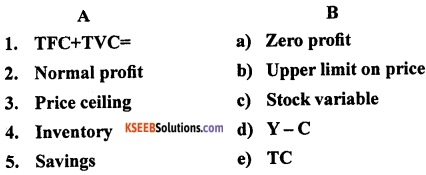1 -e; 2-a; 3 -b; 4-c; 5 -d.

IV. Answer the following questions in a sentence or a word each. (1 × 5 = 5)

Question 12.
To which side does a supply curve shift due to the technological progress?
The supply curve shifts to the right due to the technological progress.

Question 13.
What is monopoly?
It is a market with one seller or firm with many buyers.

Question 14.
Write the meaning of autonomous consumption.
The consumption which is independent of income is called as autonomous consumption.Question 15.
What are public goods?
Public goods are the goods and services provided by the government and which cannot be provided by the market mechanism. Example, roads, defence, etc.

Question 16.
What do you mean by open economy?
An open economy is one which interacts with rest of the world through various channels.

PART-B

V. Answer any NINE of the following in 4 sentences each. (2 × 9 = 18)

Question 17.
What do you mean by inferior goods? Give example.
The inferior goods are those goods for which the demand increases with the fall in income of consumer and vice-versa. That is, there will be a negative relationship between income of consumer and demand for inferior goods. Here, the income of consumer and demand move in opposite directions. Example: low-quality goods.

Question 18.
State the law of demand?
Law of demand states that other things being equal, there is a negative relation between demand for a commodity and its price. In other words, when price of the commodity increases, demand for it falls and when price of the commodity decreases, demand for it rises, other factors remaining the constant.

The law can be explained in the following manner, i.e. “Other things being equal, a fall in price leads to expansion in demand and a rise in price leads to contraction in demand”.

Question 19.
Write the meaning and formula of marginal product.
Marginal product of an input is defined as the change in output per, unit of change in the input when all other inputs are held constant. It is the additional unit of output per additional unit of variable input. It is calculated by dividing the change in output by change in input labour. The formula is
MPL = ∆TPL/∆L.

Question 20.
Mention the conditions needed for profit by a firm under perfect competition.
The following conditions needed for profit by a firm under perfect competition:

• The price P must be equal to MC
• Marginal cost must be non-decreasing at q0
• The firm to continue to produce, in the short run, price must be greater than the average variable cost and in the long run, price must be greater than the average cost.

Question 21.
Define equilibrium price and quantity.
Equilibrium price is the price at which equilibrium is reached in the market.
The equilibrium quantity is defined as the quantity which is bought and sold at equilibrium price. Therefore, price and quantity will be at equilibrium when
Qd(p*) = qs(p*)
p* denotes the equilibrium price and Qd (p*) and qs(p*) denote the market demand and market supply, respectively.Question 22.
State the meaning of average revenue and marginal revenue.
Marginal revenue of a firm is defined as the increase in total revenue for a unit increase in the firm’s output. It is obtained by dividing the change in total revenue (∆TR) by change in quantity (∆q). Thus,
MR = ∆TR/∆q.
Average revenue:
We calculate average revenue, by dividing total revenue by the quantity sold. The following formula used:
AR = TR/q.

Question 23.
What are the four factors of production? Mention their rewards.
The four factors of production are land, labour, capital and organisation. The rewards of these factors of production are as follows:

1. Land gets rent
2. Labour gets wages
3. Capital gets interest
4. Organisation gets profit.

Question 24.
Distinguish between stock and flow. Give example.

 Stock Flow • It is that quantity of economic variable which is measured at a particular point of time. • Examples are capital, inventory, wealth, foreign exchange reserves, etc. • It refers to that quantity of economic variable measured over a period of time. • Examples are net investment, salary, national income, etc.

Question 25.
Give the meaning of CRR and SLR.
The cash reserve ratio (CRR) is a certain percentage of bank deposits which a commercial bank is required to keep as cash reserves with itself.

The statutory liquidity ratio (SLR) refers to the ratio of deposits which the commercial banks have to maintain a certain percentage of their total deposits and time deposits with themselves in the form of liquid assets, as per the directions of RBI.

Question 26.
Write the meaning of excess demand and deficient demand.
If the equilibrium level of output is more than the full employment level, it is due to the fact that the demand is more than the level of output produced at full employment level. This situation is called excess demand.

If the equilibrium level of output is less than the full employment of output, it is due to fact that demand is not enough to employ all factors of production. This situation is called deficient demand.

Question 27.
Give the meaning of investment multiplier. Write its formula.
Investment multiplier is the ratio of the total increment in equilibrium value of final goods output to the initial increment in autonomous expenditure. Its formula is
Investment Multiplier = ∆Y/∆A= 1/1-c.
Where, ∆Y is the total increment in final goods output, ∆A is initial increment in autonomous expenditure and c is size of the multiplier.

Question 28.
Write the difference between public provision and public production.
The difference between public provision and public production are as follows:

 Public Provision Public Production • A set of facilities financed by the government through its budget. • These are used without any direct payment. Examples are free education, mid-day meals, etc. • When the goods produced directly by the government, it is called public production. • These are used with direct payment. Examples are electricity, water supply, etc.Question 29.
Mention the three linkages of open economy.
The three linkages of open economy are as follows:

Question 30.
What is the difference between current account and capital account?
The difference between current account and capital account are as follows:

 Current Account Capital Account • It is the record of trade in goods and services, and transfer payments. • It consists of factor and non-factor incomes apart from gifts, remittances, and grants. • It is the record of all international transactions of assets. • It includes money, stocks, bonds, government debt, etc.

PART-C

VI. Answer any SEVEN of the following questions in 12 sentences each. (4 × 7 = 28)

Question 31.
Briefly explain the production possibility frontier.
The production possibility frontier is a graphical representation of the combinations of two commodities (cotton and wheat) that can be produced when the resources of the economy are fully utilized. It is also called as production possibility curve (PPC) also known as transformation curve.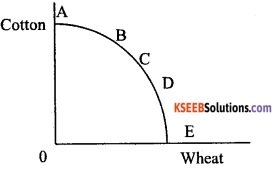It gives the combinations of cotton and wheat that can be produced when the resources of the economy are fully utilized. This can be graphically represented as follows.

As per the above graph, the points lying strictly below the production possibility curve represents a combination of cotton and wheat that will be produced when all or some of the resources are either underemployed or are utilized in a wasteful fashion.

Question 32.
Explain the in difference map with the diagram.
A family of indifference curves is called as in difference map. It refers to a set of indifference curves for two commodities showing different levels of satisfaction. The higher indifference curves show higher level of satisfaction and lower indifference curve represent lower satisfaction. A rational consumer always chooses more of that product which offers him a higher level of satisfaction which is represented in higher indifference curve. It is also called ‘monotonic preferences’.

The consumer’s preferences over all the bundles . can be represented by a family of indifference curves as shown in the following diagram.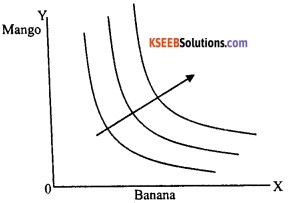In the given diagram, we see the group of three indifference curves showing different levels of satisfaction to the consumer. The arrow indicates that bundles on higher indifference curves are preferred by the consumer to the bundles on lower indifference curves.

Question 33.
Explain isoquant with the help of a diagram.
An isoquant is the set of all possible combinations of the two inputs that yield the same maximum possible level of output. Each isoquant represents a particular level of output and is labelled with that amount of output. It is just an alternative way of representing the production function.
The concept of isoquant can be explained with the help of following diagram: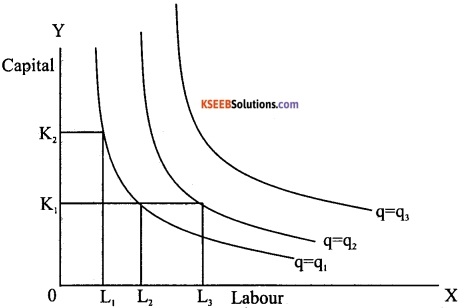The given diagram generalizes the concept of isoquant. In the above diagram, labour is measured in OX axis and capital is measured in OY axis. There are 3 isoquants for the three output levels, viz. q=q1 q=q2 and q=q3. Two input combinations (L1, K2) and (L2, K1) give us the same level of output q1.

If we fix capital at K1 and increase labour to L3, output increases and we reach a higher isoquant q=q2. When marginal products are positive, with greater amount of one input, the same level of output can be produced only using lesser amount of the other. Therefore, isoquant curves slope downwards from left to right (negatively sloped).

Question 34.
Write a brief note on returns to scale.
The returns to scale can happen only in the long run as both the factors (labour and capital) can be changed. One special case, in the long run, occurs when both factors are increased by the same proportion or factors are scaled up.

a. Constant returns to scale:
When a proportional increase in all inputs results in an increase in output by the same proportion, the production function is said display constant returns to scale.

b. Increasing returns to scale:
When proportional increase in all inputs results in an increase in output by a larger proportion, the production function is said to display increasing returns to scale.

c. Decreasing returns to scale:
When a proportional increase in all inputs results in an increase in output by a smaller proportion, the production function is said to display decreasing returns to scale.

For example, if in a production process, all inputs get doubled. As a result, if the output gets doubled, the production function exhibits constant returns to scale, if output is less than doubled, exhibits decreasing returns to scale and if it is more than doubled, exhibits increasing returns to scale.Question 35.
Explain the determinants of a firm’s supply curve.
A firm’s marginal cost curve is a part of its marginal cost curve. Any factor that affects a firm’s marginal cost curve is a determinant of its supply curve. Following are the two factors determining a firm’s supply curve:

a. Technological progress:
The organisational innovation by the firm leads to more production of output. That means to produce a given level of output, the organisational innovation allows the firm to use fewer units of inputs. It is expected that this will lower the firm’s marginal cost at any level of output, i.e. there is a rightward shift of the MC curve. As the firm’s supply curve is essentially a segment of the MC curve, technological progress shifts the supply curve of the firm to the right. At any given market price, the firm now supplies more quantity of output.

b. Input prices:
A change in the prices of factors of production (inputs) also influences a firm’s supply curve. If the price of input (e.g. wage) increases, the cost of production also increases. The consequent increase in the firm’s average cost at any level of output is usually accompanied by an increase in the firm’s marginal cost at any level of output, which leads to an upward shift of the MC curve. That means the firm’s supply curve shifts to the left and the firm produces less quantity of output.

Question 36.
Write a short note on profit maximization of a firm under the following conditions

1. P=MC
2. MC must be none decreasing at q0.

A firm always wishes to maximize its profit. The firm would like to identify the quantity q0, the firm’s profits are less at q0 For profits to be maximum, the following conditions must hold at q0.

1. The price P must equal MC (P = MC):
Profit is the difference between total revenue and total cost. Both total revenue and total cost increase as output increases. As long as the change in total revenue is greater than the change in total cost, profits will continue to increase.

The change in total revenue per unit increase in output is the marginal revenue and the change in total cost per unit increase in output is the marginal cost.

Therefore, we can conclude that as long as marginal revenue is greater than marginal cost, profits are increasing and as long as marginal revenue is less than marginal cost, profits will fall. It follows that for profits to be maximum, marginal revenue should be equal to marginal cost.

For the perfectly competitive firm, we have established that the MR=P. So the firm’s profit-maximizing output becomes the level of output at which P = MC.

2. Marginal cost must be non-decreasing at q0:
It means that the marginal cost curve cannot slope downwards at the profit-maximizing output level. This can be explained with the help of diagram: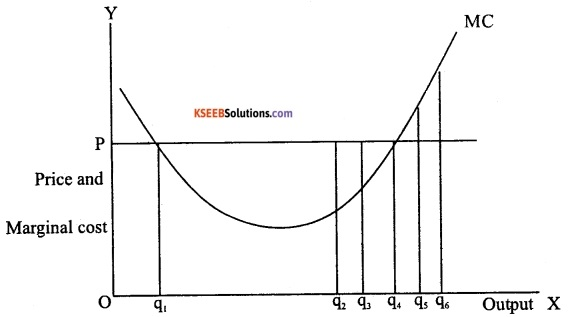In the given diagram, at output levels q1 and q4 the market price is equal to the marginal cost. However, at the output level q1, the marginal cost curve is downward sloping. The q1 is not profit-maximizing output level.

If we observe all output levels left to the q1 the market price is lower than the marginal cost. But the firm’s profit at an output level slightly smaller than q1 exceeds that corresponding to the output level q1 Therefore, q1 cannot be a profit-maximizing output level.

Question 37.
Explain the role of the government (state) and household sector in both developed and developing countries.
1. Role of government:
In both the developed and developing countries, apart from capitalist sector, there is the institution of state. The role of the state includes framing laws, enforcing them and delivering justice. The state here refers to the government which performs various developmental functions for the society as whole.

It undertakes production, apart from imposing taxes and spending money on building public infrastructure, running schools, providing health services, etc. These economic functions of the state have to be taken into account when we want to describe the economy of the country.

2. Role of household sector:
By household we mean a single individual who takes decisions relating to her own consumption or a group of individuals for whom the decisions relating to consumption are jointly determined. Households consist of people. These people work in firms as workers and earn wages.

They are the one who work in government departments and earn salaries or they are the owners of firms and earn profits. Therefore, the market in which the firms sell their products could not have been functioning without the demand coming from the households. Further, they also earn rent by leasing land or earn interest by lending capital.

Question 38.
Write a note on externalities.
An externality is a cost or benefit conferred upon second or third parties as a result of acts of individual production and consumption. But the cost or benefit of an externality cannot be measured in money terms because it is not included in market activities.

In other words, externalities refer to the benefits or harm a firm or an individual causes to another for which they are not paid or penalized. They do not have any market in which they can be bought and sold. There are two types of externalities viz.,

1. Positive externalities
2. Negative externalities.

For example, let us imagine that there is chemical fertilizer industry. It produces the chemical fertilizers required for agriculture. The output of the industry is taken for counting GDP of an economy. This is positive externality.

While carrying out the production, the chemical fertilizer industry may also be polluting the nearby river. This may cause harm to the people who use the water of the river. Hence their health will be affected. Pollution also may kill fish and other organisms of the river. As a result, the fishermen of the river may lose their livelihood. Such harmful effects that the industry is inflicting on others, for which it will not bear any cost are called negative externalities.

Question 39.
Write the meaning of transaction motive and speculative motive of demand for money and liquidity trap.
a. Transaction motive:
Transaction motive demand for money refers to holding money to carryout transactions. If we receive our income weekly and make payments on the first day of every week, we need not hold any cash balance throughout the rest of the week. But our expenditure patterns do not normally match our receipts. People earn incomes at discrete points in time and spend it continuously throughout the interval.
The transaction demand for money is represented as follows:
MdT = k. T
Where, T is the total value of transactions in the economy over unit period and k is a position fraction.

b. Speculative motive:
Some people hold cash to invest on shares, debentures, gold, immovable properties, etc. The speculative demand for money refers to the demand for money that people hold as idle cash to speculate with the aim of earning capital gains and profits. The speculative demand for money can be written as follows:
$$M_{s}^{d}=\frac{r_{\max }-r}{r-r_{\min }}$$
Where, r is the market rate of interest and rmax and rmin are the upper and lower limits of r, both positive constants. It clearly states that as r decreases from rmax to rmin, the value of speculative demand for money decreases from zero to infinity.Question 40.
Write a note on balance of trade.
Balance of trade is the difference between the value of exports and value of imports of goods of a country in a given period of time. Export of goods is entered as a credit item in balance of trade. Import of goods is entered as a debit item in balance of trade. It is also called as trade balance. Balance of that is said to be in balance when exports of goods are equal to the imports of goods, i.e. balanced balance of trade.

Surplus balance of trade arises if country’s exports of goods are more than its imports. Deficit balance of trade arises if a country’s imports of goods are more than its exports. Balance of trade is narrow concept and it may not show the international economic position of an economy. It gives partial picture of international transactions and it is less reliable. It does not include net invisibles, i.e. the difference between the value of exports and value of imports of invisibles (services) of a country in a given period of time.

Question 41.
Explain the law of diminishing marginal utility with the help of a table and diagram.
One of the most important propositions of the cardinal utility approach to demand was the Law of Diminishing Marginal Utility. German Economist Gossen was the first to explain it. Therefore, it is called Gossen’s First Law.

According to Alfred Marshall, “The additional benefit which a person derives from a given increase of a stock of a thing diminishes, other things being equal, with every increase in the stock that he already has”.

This law simply tells us that, we obtain less and less utility from the successive units of a commodity as we consume more and more of it.

Assumptions of the Law of DMU

1. Uniform quality and size of the commodity:
The successive units of the commodity should not differ in any way either in quality or size.

2. Suitable quantity of consumption:
The Commodity units should not be very small, e.g. milk should be in glasses and not in spoons.

3. Consumption within the same time:
Consumption must be continuous. There should not be so much difference in time between the consumption of successive units.

4. No change in the price of the commodity or its substitutes:
The law is based on the assumption that the commodity’s price is not changed with successive units. The price of the substitutes is also kept at the same level.

5. Utility can be measured in cardinal numbers, i.e. 1, 2, 3, 4, …….

6. Consumer must be rational, i.e. every consumer wants to maximize his satisfaction.

The basis of this law is that every want needs to be satisfied only up to a limit. After this limit is reached the intensity of our want becomes zero. It is called complete satisfaction of the want. Therefore, we consume more and more units of a commodity to satisfy our need, the intensity of our want for it becomes less and less.

Therefore, the utility obtained from the consumption of every unit of the commodity is less than that of the units consumed earlier. This can be explained with the help of the following table.

 Units of Apples Total Utility (TU) Marginal Utility (MU) 1 30 30 2 50 20 3 65 15 4 75 10 5 80 5 6 82 2 7 82 0 8 80 -2

Suppose a man wants to consume apples and is hungry. In this condition, if he gets one apple, he has very utility for it. Let us say that the measurement of this utility is equal to 30 utils. Having eaten the first he will not remain so hungry as before. Therefore, if he consumes the second apple he will have a lesser amount of utility from the second apple even if it was exactly like first one.

The utility he got from the second apple equals 20 units, the third, fourth, fifth and sixth apples give him utility equal to 15, 10, 5 and 2 units respectively. Now, if he is given the seventh apple he has no use for it. That means the utility of the seventh apple to the consumer is zero.

It is just possible that if he is given the eighth apple for consumption, it may harm him. Here the utility will be
negative, i.e. -2. Therefore, we are clear that the additional utility of the successive apples to the consumer goes on diminishing as he consumes more and more of it.

The Law of Diminishing Marginal Utility can be explained with the help of the following diagram.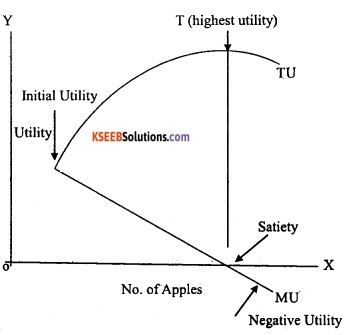In the diagram, the horizontal axis shows the units of apples and the vertical axis measures the MU and TU obtained from the apple units. The total utility curve will be increasing in the beginning and later falls. The marginal utility curve is falling from left down to the right clearly tells us that the satisfaction derived from the successive consumption of apples is falling.

The marginal utility of the first apple is known as initial utility. It is 30 utils. The marginal utility of the seventh apple is zero. Therefore, this point is called the satiety point. The marginal utility of the eighth apple is -2. So, it is called negative utility and lies below the X-axis.

PART-D

VII. Answer any Six of the following questions in 20 sentences each. (6 × 4 = 24)

Question 42.
Explain the market equilibrium with the fixed number of firms with the help of diagram.
Under perfect competition, market is said to be in equilibrium when quantity demanded is equal to the quantity supplied. Here, with the help of market demand curve and market supply curve, we will determine where the market will be in equilibrium when the number of firms is fixed.
This can be illustrated with the help of the following diagram.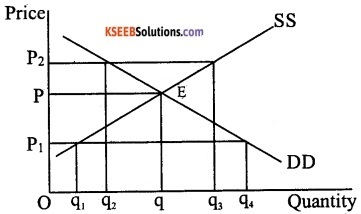The given diagram illustrates equilibrium for a perfectly competitive market with a fixed number of firms. SS is market supply curve and DD is market demand curve. The market supply curve SS shows how much of the commodity firms would wish to supply at different prices and the demand curve DD tells us how much of the commodity, the consumer would be willing to purchase at different prices.

At point E, the market supply curve intersects the market demand curve which denotes that quantity demanded is equal to quantity supplied. At any other point, either there is excess supply or there is excess demand.

OP is the equilibrium price and Oq is the equilibrium quantity. If the price is P1, the market supply is q1 and market demand is q4. Therefore, there is excess demand in the market equal to q1q4 Some consumers who are either unable to obtain the commodity at all or obtain it in insufficient quantity will be willing to pay more than P1 The market price would tend, to increase.

All other things remaining constant, when the price increases the demand falls and quantity supplied rises. The market moves towards equilibrium where quantity demanded is equal to quantity supplied. It happens at P where supply decisions match demand decisions.

If the price is P2, the market supply q3 will exceed the market demand q2 which leads to excess supply equal to q2q3 Some firms will not be able to sell quantity they want to sell. Therefore, they will lower their price.

All other things remaining constant, when the price falls, quantity, demanded rises and quantity supplied falls to equilibrium price P where the firms are able to sell their desired output as market demand equals market supply at P. So, the P is the equilibrium price and the corresponding quantity q is the equilibrium quantity.Question 43.
Explain the short-run equilibrium of a monopolist firm, when the cost of production is positive by using TR and TC curves with the help of diagram.
The short-run equilibrium of a monopolist firm, when the cost of production is positive by using TR and TC curves can be explained with the help of diagram as follows: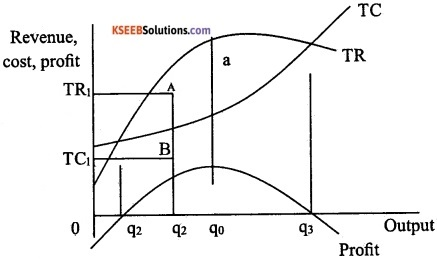In the given diagram, total cost, total revenue, and profit curves are drawn. The profit received by the firm equals the total revenue minus the total cost. In the diagram, if quantity q1 is produced, the total revenue is TR1 and total cost is TC1. The difference TR1 – TC1 is the profit received. The same is depicted by the length of the line segment AB, i.e. the vertical distance between the TR and TC U curves at q1 level of output.

If the output level is less than q2, the TC curve lies above the TR curve, i.e. TC is greater than TR and therefore profit is negative and the firm makes losses. The same situation exists for output levels greater than q3. Hence, the firm can make positive profits only at output levels between q2 and q3.

where TR curve lies above the TC curve. The monopoly firm will chose that level of output which maximizes its profit. This would be level of output for which the vertical distance between TR and TC is maximum and TR is above the TC, i.e. TR – TC is maximum. This occurs at the output level q0.

Question 44.
Explain the macroeconomic identities.
The macroeconomic identities are as follows:

1. Gross domestic product (GDP):
Gross domestic product measures the aggregate production of final goods and services taking place within the domestic economy during a year. But the whole of it may not accrue to the citizens of the country. It includes GDP at market prices and GDP at factor cost.

GDP at market price is the market value of all final goods and services produced within a domestic territory of a country measured in a year. Here everything is valued at market prices. It is obtained as follows:
GDPMP = C + I + G + X – M
GDP at factor cost is gross domestic product at market prices minus net indirect taxes. It measures money value of output produced by the firms within the domestic boundaries of a country in a year.
GDPFC = GDPMP – NIT.

2. Gross national product:
It refers to all the economic output produced by a nation’s normal residents, whether they are located within the national boundary or abroad. It is defined as GDP plus factor income earned by the domestic factors of production employed in the rest of the world minus factor income earned by the factors of production of the rest of the world employed in the domestic economy. Therefore,
GNP = GDP + Net factor income from abroad.

3. Net national product (NNP):
A part of the capital gets consumed during the year due to wear and tear. This wear and tear is called depreciation. If we deduct depreciation from GNP the measure of aggregate income that we obtain is called net national product. We get the value of NNP evaluated at market prices. So,
NNP = GNP – Depreciation.

4. Net national product (NNP) at factor cost:
The NNP at factor is the sum of income earned by all factors in the production in the form of wages, profits, rent, and interest, etc. belong to a country during a year. It is also known as national income. We need to add subsidies to NNP and deduct indirect taxes from NNP to obtain NNP at factor cost.
NNPFC = NNP at market prices – indirect taxes + subsidies

5. Personal income (PI):
It refers to the part of national income (NI) which is received by households. It is obtained as follows:
PI = NI – Undistributed Profits -Net interest payments made by the households – Corporate tax + Transfer payments to the households from the government and firms.

6. Personal disposable income (PDI):
If we deduct the personal tax payments (income tax) and non-tax payments (fines, fees) from personal income, we get PDI. Therefore,
PDI = PI – Personal tax payments – Non-tax payments.

Question 45.
Explain the functions of money. How does money overcome the shortcomings of a barter system?
The functions of money are broadly classified as follows:

1. Primary functions
2. Secondary functions
3. Contingent functions.

I. Primary functions: The primary functions of money are as follows:

a. Medium of exchange:
Money plays an important role as a medium of exchange. It facilitates exchange of goods for money. It has solved the problems of barter system. Barter exchanges become extremely difficult in a large economy because of the high costs people would have to incur looking for’suitable persons to exchange their surpluses. It helps the people to sell in one place and buy-in another place. Money has widened the scope of market transactions. Money has become a circulating material between buyers and sellers.

b. Measure of value/unit of account:
The money acts as a common measure of value. The values of all goods and services can be expressed in terms of money. As a measure of value, money performs following functions:

1. The value of all goods and services measured and expressed in terms of the money
2. Rate of exchange of goods and services expressed in money
3. Facilitates the maintenance of accounts
4. It facilitates price mechanism
5. It makes goods and services comparable in terms of price.

For instance, when we say that the value of a book is Rs.500 we mean that the book can be exchanged for 500 units of money where a unit of money is rupee in this case. If the price of a pencil is Rs.5 and that of a pen is Rs.10 we can calculate the relative price of a pen with respect to a pencil, i.e. a pen is worth 10/5=2 pencils.

II. Secondary functions: The secondary functions of money are as follows:

a. Store of value:
People can save part of their present income and hold the same for future. Money can be stored for precautionary motives needed to overcome financial stringencies. Money solves one of the deficiencies of barter system, i.e. difficulty to cany forward one’s wealth under the barter system.

For instance, we have an endowment of wheat which we do not wish to consume today entirely. We may regard this stock of surplus wheat as an asset which we may wish to consume or even sell-off”, for acquiring other commodities at some future date. But wheat is a perishable item and cannot be stored beyond a certain period.

Also, holding the stock of rice required a lot of space. We may have to spend considerable time and resources looking for people with a demand for wheat when we wish to exchange our stock for buying other commodities. This problem can be solved if we sell our wheat for money. Money is not perishable land its storage costs are also less.

b. Standard of deferred payments:
All the credit transactions are expressed in terms of money. The payment can be delayed or postponed. So, money can be used for delayed settlement of dues or financial commitments.

c. Transfer of value:
Money acts as a transfer of value from person to person and from place to place. As a transfer of value, money helps us to buy goods, properties or anything from any part of the country or the world. Further, money earned in different places can be brought or transferred to anywhere in the world.

III. Contingent functions of money: Other than primary and secondary functions, money also performs other functions which are as follows:

a. Basis of credit:
Money serves as a basis of the credit. The modem credit system exists only because of existence of money.

b. Distribution of national income:
Money helps in distribution of national income. The reward paid to factors of production in the form of rent, wages, interest, and profit are nothing but the distribution of national income at factor prices.

c. Provides liquidity and uniformity:
Money provides liquidity to all kinds of assets both moveable and immovable. Money can be converted into any type of asset and all assets can be converted into money.

d. Helps in consumers’ and producers’ equilibrium:
All goods and services are expressed in terms of money. The consumer attains equilibrium when the price of a product is equal to his marginal utility. Similarly, the producers reach equilibrium if they get maximum satisfaction. Both consumers and producers try to achieve equilibrium with the help of money.Question 46.
Explain the classification of receipts.
The government budget consists of revenue budget and capital budget. Both the budgets have receipts viz., revenue receipts and capital receipts.

I. Revenue receipts:
Revenue receipts are those receipts which do not lead to a claim on the government. They include the following:

1. Tax revenue.
2. Non-tax revenue.

1. Tax revenues:
These are the important component of revenue receipts. Tax revenue consists of direct tax and indirect taxes. The direct tax includes income tax, corporate tax and indirect tax includes excise duty (tax on production of goods in the country), customs duties (tax on exports and imports) and service tax (GST-goods and services tax has been introduced in place of indirect taxes from 1st July 2017). Other direct taxes like wealth tax and gift tax have never brought in large amount of revenue and thus they are called as paper taxes.

2. Non-tax revenue:
The non-tax revenue of the central government consists of the following:

• Interest receipts on account of loans by the central government
• Dividends and profits on investments made by the government
• Fees and other receipts for services rendered by the government
• Grants-in-aid from foreign countries and international organizations.

II. Capital receipts:
All those receipts of the government which create liability or reduce financial assets are termed as capital receipts. The government receives money by way of loan or from the sale of its assets. Loans have to be repaid to the agencies from whom the government has borrowed. Thus it creates liability. Sale of government assets like sale of shares in public sector undertakings (disinvestment) reduces the total amount of financial assets of the government.

When government takes fresh loans it means that it has to be returned with interest. Similarly, when government sells an asset it means that in future its earnings from that asset will disappear. Thus, these receipts can be debt creating or non-debt creating.

Question 47.
Briefly explain the foreign exchange market with fixed exchange rates with the help of a diagram.
Under fixed exchange rate system, the government decides the exchange rate at a particular level. The foreign exchange market with fixed exchange rates can be explained with the help of following diagram.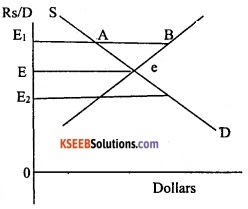In the given diagram, the market-determined exchange rate is e where demand and supply intersect. However, if the government wants to encourage exports for which it needs to make rupee cheaper for foreigners it would do so by fixing a higher exchange rate say, Rs.70 per dollar from the current exchange rate of Rs.65 per dollar.

Thus, the new exchange rate set by the government is E1 where E1 is greater E. At this exchange rate, supply of dollars exceeds the demand for dollars. The RBI intervenes to purchase the dollars for rupees in the foreign exchange market in order to absorb this excess supply which has been marked as AB in the diagram.

Thus, by interfering, the government can maintain any exchange rate in the economy. If the government wants to set an exchange rate at a level E2 there would be an excess demand for dollars, the government would have to withdraw dollars from its past holds of dollar. If the government fails to do so, it will encourage black market transactions.

Question 48.
Briefly explain the expenditure method of measuring GDP.
Expenditure method is the alternative way to calculate the GDP by looking at the demand side of the products. Here, the aggregate value of the output in the economy by expenditure method will be calculated in the following way.

In this method, we add the final expenditures that each firm makes. Final expenditure is that part of expenditure which is undertaken not for intermediate purposes. If the baker buys Rs. 50 worth of wheat from the farmers is considered as intermediate good and the final expenditure received by the baker is 200. Then the aggregate value of output of the economy is Rs. 200 + Rs. 50 = Rs. 250.

Let us assume that firm ‘i’ makes the final expenditure on the following accounts:

1. Final consumption expenditures on the goods and services by households denoted as Ci.
2. Final investment expenditure incurred by the firms on capital goods denoted as Ii.
3. The expenditure that the government makes on the final goods and services produced by the firm, denoted as Gi.
4. The export revenues that firm i earns by selling its goods and services abroad denoted as Xi.

Now the total final consumption, investment, government and export expenditures received by the firm i. Now GDP according to the expenditure method is expressed as follows:

GDP = ΣNi-1RVi = C + I + G + X-M

ΣNi-1 RVi is the sum of final consumption C, investment is I, government is G and exports is X expenditures (M is imports) received by all the firms in the economy.

PART-E

VIII. Answer any two of the following project-oriented questions. (5 × 2=10)

Question 49.
A consumer wants to consume two goods. The price of bananas is Rs.4 and price of mangoes is Rs.5. The consumer income is Rs.20.

1. How many bananas can she consume if she spend her entire income on that good?
2. How many mangoes can she consume if she spend her entire income on that good?
3. Is the slope of budget line is downward or upward?
4. Are the bundles on the budget line equal to the consumers’ income or not?
5. If you want to have more of banana, you have to give up mangoes. Is it true?

1. 5 Bananas (20/4).
2. 4 Mangoes (20/5).
3. Slope of budget line is downward.
4. Yes, the bundles on the budget line are equal to the consumer’s income.
5. True, if we want to have more of banana we have to give up mangoes.

Question 50.
From the following data calculate personal income and personal disposable income.

 (a) Net domestic product at factor cost 8,000 (b) Net factor income from abroad 200 (C) Undisbursed profit 1,000 (d) Corporate tax 500 (e) Interest received by households 1,500 (f) Interest paid by households 1,200 (g) Transfer income 300 (h) Personal tax 500

Personal income = Net domestic product at factor cost + Net factor income from abroad + (Interest received by households – Interest paid by households) + Transfer income – Corporate tax – Undisbursed profit.
Therefore, – PI = 8000 + 200 + (1500 – 1200) + 300 – 500 -1000
= 8000 + 200 + 300+300-500-1000
= 7300
Personal Disposable Income = Personal Income – Personal Tax
Therefore PDI = 7300 – 500
= 6800.Question 51.
Name the currencies of any five countries of the following USA, UK, Germany, Japan, China, Argentina, UAE, Bangladesh, Russia.